# 9.4 The Second and Third Laws of Thermodynamics

Learning Objectives

By the end of this section, you will be able to:

• State and explain the second and third laws of thermodynamics
• Calculate entropy changes for phase transitions and chemical reactions under standard conditions

## The Second Law of Thermodynamics

In the quest to identify a property that may reliably predict the spontaneity of a process, a promising candidate has been identified: entropy. Processes that involve an increase in entropy of the system (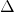S > 0) are very often spontaneous; however, examples to the contrary are plentiful. By expanding consideration of entropy changes to include the surroundings, we may reach a significant conclusion regarding the relation between this property and spontaneity. In thermodynamic models, the system and surroundings comprise everything, that is, the universe, and so the following is true: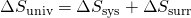To illustrate this relation, consider again the process of heat flow between two objects, one identified as the system and the other as the surroundings. There are three possibilities for such a process:

1. The objects are at different temperatures, and heat flows from the hotter to the cooler object. This is always observed to occur spontaneously. Designating the hotter object as the system and invoking the definition of entropy yields the following: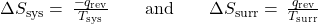The magnitudes of −qrev and qrev are equal, their opposite arithmetic signs denoting loss of heat by the system and gain of heat by the surroundings. Since Tsys > Tsurr in this scenario, the entropy decrease of the system will be less than the entropy increase of the surroundings, and so the entropy of the universe will increase: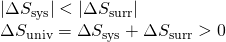2. The objects are at different temperatures, and heat flows from the cooler to the hotter object. This is never observed to occur spontaneously. Again designating the hotter object as the system and invoking the definition of entropy yields the following: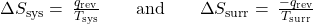The arithmetic signs of qrev denote the gain of heat by the system and the loss of heat by the surroundings. The magnitude of the entropy change for the surroundings will again be greater than that for the system, but in this case, the signs of the heat changes (that is, the direction of the heat flow) will yield a negative value forSuniv. This process involves a decrease in the entropy of the universe.

3. The objects are at essentially the same temperature, TsysTsurr, and so the magnitudes of the entropy changes are essentially the same for both the system and the surroundings. In this case, the entropy change of the universe is zero, and the system is at equilibrium.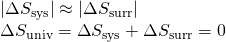These results lead to a profound statement regarding the relation between entropy and spontaneity known as the second law of thermodynamics: all spontaneous changes cause an increase in the entropy of the universe. A summary of these three relations is provided in (Figure 9.4.1).

Figure 9.4.1 – The Second Law of ThermodynamicsSuniv > 0 spontaneousSuniv < 0 nonspontaneous (spontaneous in opposite direction)Suniv = 0 at equilibrium

For many realistic applications, the surroundings are vast in comparison to the system. In such cases, the heat gained or lost by the surroundings as a result of some process represents a very small, nearly infinitesimal, fraction of its total thermal energy. For example, combustion of a fuel in air involves transfer of heat from a system (the fuel and oxygen molecules undergoing reaction) to surroundings that are infinitely more massive (the earth’s atmosphere). As a result, qsurr is a good approximation of qrev, and the second law may be stated as the following: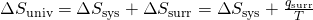We may use this equation to predict the spontaneity of a process.

Activity 9.4.1 – Will Ice Spontaneously Melt?

The entropy change for the process: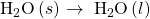is 22.1 J/K and requires that the surroundings transfer 6.00 kJ of heat to the system. Is the process spontaneous at −10.00 °C? Is it spontaneous at +10.00 °C?

## Solution

We can assess the spontaneity of the process by calculating the entropy change of the universe. IfSuniv is positive, then the process is spontaneous. At both temperatures,Ssys = 22.1 J/K and qsurr = −6.00 kJ.

At −10.00 °C (263.15 K), the following is true: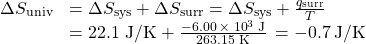Suniv < 0, so melting is nonspontaneous (not spontaneous) at −10.0 °C.

At 10.00 °C (283.15 K), the following is true: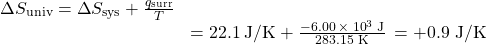Suniv > 0, so melting is spontaneous at 10.00 °C.

Using this information, determine if liquid water will spontaneously freeze at the same temperatures. What can you say about the values of Suniv?

Entropy is a state function, soSfreezing = −Smelting = −22.1 J/K and qsurr = +6.00 kJ. At −10.00 °C spontaneous, +0.7 J/K; at +10.00 °C nonspontaneous, −0.9 J/K.

## The Third Law of Thermodynamics

The previous section described the various contributions of matter and energy dispersal that contribute to the entropy of a system. With these contributions in mind, consider the entropy of a pure, perfectly crystalline solid possessing no kinetic energy (that is, at a temperature of absolute zero, 0 K). This system may be described by a single microstate, as its purity, perfect crystallinity and complete lack of motion means there is but one possible location for each identical atom or molecule comprising the crystal (W = 1). According to the Boltzmann equation, the entropy of this system is zero: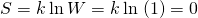This limiting condition for a system’s entropy represents the third law of thermodynamics: the entropy of a pure, perfect crystalline substance at 0 K is zero.

Careful calorimetric measurements can be made to determine the temperature dependence of a substance’s entropy and to derive absolute entropy values under specific conditions. Standard entropies (S°) are for one mole of substance under standard conditions (a pressure of 1 bar and a temperature of 298.15 K; see details regarding standard conditions in the thermochemistry chapter of this text). The standard entropy change (S°) for a reaction may be computed using standard entropies as shown below: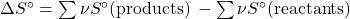where ν represents stoichiometric coefficients in the balanced equation representing the process. For example,S° for the following reaction at room temperature: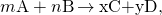is computed as: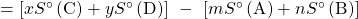A partial listing of standard entropies is provided in (Table 9.4.1), and additional values are provided in Appendix G. The example exercises that follow demonstrate the use of S° values in calculating standard entropy changes for physical and chemical processes.

 Substance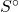(J mol−1 K−1) carbon C(s, graphite) 5.740 C(s, diamond) 2.38 CO(g) 197.7 CO2(g) 213.8 CH4(g) 186.3 C2H4(g) 219.5 C2H6(g) 229.5 CH3OH(l) 126.8 C2H5OH(l) 160.7 hydrogen H2(g) 130.57 H(g) 114.6 H2O(g) 188.71 H2O(l) 69.91 HCI(g) 186.8 H2S(g) 205.7 oxygen O2(g) 205.03

Activity 9.4.2 – Determination ofS°

Calculate the standard entropy change for the following process: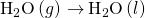## Solution

Calculate the entropy change using standard entropies as shown above: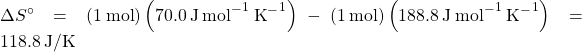The value forS° is negative, as expected for this phase transition (condensation), which the previous section discussed.

Calculate the standard entropy change for the following process: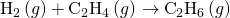−120.6 J/K

Activity 9.4.3 – Determination ofS°

Calculate the standard entropy change for the combustion of methanol, CH3OH: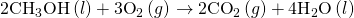## Solution

Calculate the entropy change using standard entropies as shown above: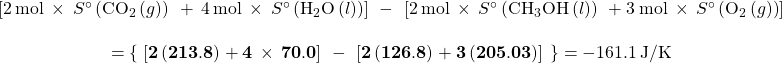Calculate the standard entropy change for the following reaction: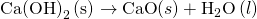24.7 J/K

# Key Concepts and Summary

The second law of thermodynamics states that a spontaneous process increases the entropy of the universe, Suniv > 0. IfSuniv < 0, the process is nonspontaneous, and ifSuniv = 0, the system is at equilibrium. The third law of thermodynamics establishes the zero for entropy as that of a perfect, pure crystalline solid at 0 K. With only one possible microstate, the entropy is zero. We may compute the standard entropy change for a process by using standard entropy values for the reactants and products involved in the process.

## Key Equations

•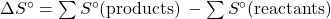•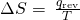•Suniv =Ssys +Ssurr
•End of Chapter Exercises

(1) What is the difference betweenS andS° for a chemical change?

(2) Calculate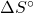for the following changes.

(2a)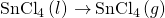(2b)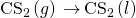(2c)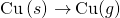(2d)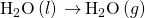(2e)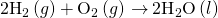(2f)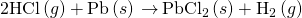(2g)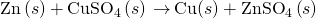Solution

(a) 107 J/K; (b) −86.4 J/K; (c) 133.2 J/K; (d) 118.8 J/K; (e) −326.6 J/K; (f) −171.9 J/K; (g) −7.2 J/K

(3) Determine the entropy change for the combustion of liquid ethanol, C2H5OH, under the standard conditions to give gaseous carbon dioxide and liquid water.

(4) Determine the entropy change for the combustion of gaseous propane, C3H8, under the standard conditions to give gaseous carbon dioxide and water.

Solution

100.6 J/K

(5) “Thermite” reactions have been used for welding metal parts such as railway rails and in metal refining. One such thermite reaction is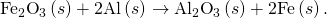Is the reaction spontaneous at room temperature under standard conditions? During the reaction, the surroundings absorb 851.8 kJ/mol of heat.

(6) Using the relevant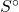values listed in Appendix G, calculatefor the following changes:

(6a)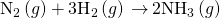(6b)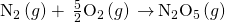Solution

(a) −198.1 J/K; (b) −348.9 J/K

(7) From the following information, determinefor the following: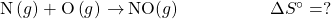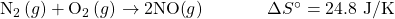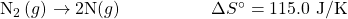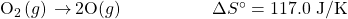(8) By calculatingSuniv at each temperature, determine if the melting of 1 mole of NaCl(s) is spontaneous at 500 °C and at 700 °C.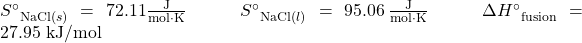(9) What assumptions are made about the thermodynamic information (entropy and enthalpy values) used to solve this problem?

Solution

AsSuniv < 0 at each of these temperatures, melting is not spontaneous at either of them. The given values for entropy and enthalpy are for NaCl at 298 K. It is assumed that these do not change significantly at the higher temperatures used in the problem.

Use the standard entropy data in Appendix G to determine the change in entropy for each of the following reactions. All the processes occur at the standard conditions and 25 °C.

(9a)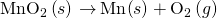(9b)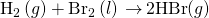(9c)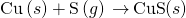(9d)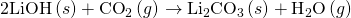(9e)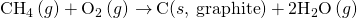(9f)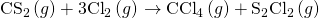(10) Use the standard entropy data in Appendix G to determine the change in entropy for each of the reactions listed in Q9. All the processes occur at the standard conditions and 25 °C.

Solution

(a) 2.86 J/K; (b) 24.8 J/K; (c) −113.2 J/K; (d) −24.7 J/K; (e) 15.5 J/K; (f) 290.0 J/K

## Glossary

all spontaneous processes involve an increase in the entropy of the universe

entropy for one mole of a substance at 1 bar pressure; tabulated values are usually determined at 298.15 K

change in entropy for a reaction calculated using the standard entropies

entropy of a perfect crystal at absolute zero (0 K) is zero